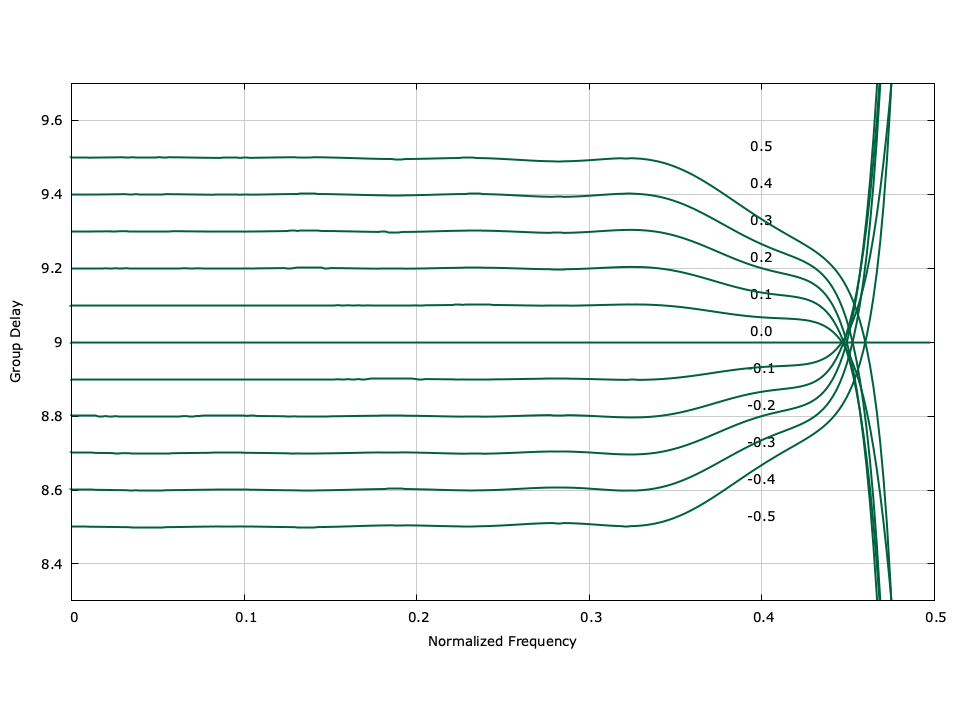# Finite Impulse Response Farrow Filter (firfarrow)

API Keywords: firfarrow

liquid implements non-recursive Farrow filters using the firfarrow family of objects. The Farrow structure is convenient for varying the group delay of a filter. The filter coefficients themselves are not stored explicitly, but are represented as a set of polynomials each with an order $$Q$$ . The coefficients can be computed dynamically from the polynomial by arbitrarily specifying the fractional sample delay $$\mu$$ . Listed below is the full interface to the firfarrow family of objects. While each method is listed for firfarrow_crcf , the same functionality applies to firfarrow_rrrf .

• firfarrow_crcf_create(N,Q,fc,As) creates a firfarrow object with $$N$$ coefficients using a polynomial of order $$Q$$ with a cutoff frequency $$f_c$$ and as stop-band attenuation of $$A_s$$ dB.
• firfarrow_crcf_destroy(q) destroy object, freeing all internally-allocated memory.
• firfarrow_crcf_clear(q) clear filter internal memory buffer. This does not reset the delay.
• firfarrow_crcf_print(q) prints the filter's internal state to stdout .
• firfarrow_crcf_push(q,x) push a single sample $$x$$ into the filter's internal buffer.
• firfarrow_crcf_set_delay(q,mu) set fractional delay $$\mu$$ of filter.
• firfarrow_crcf_execute(q,*y) computes the output sample, storing the result in $$y$$ .
• firfarrow_crcf_get_length(q) returns length of the filter (number of taps)
• firfarrow_crcf_get_coefficients(q,*h) returns the internal filter coefficients, storing the result in the output vector $$\vec{h}$$ .
• firfarrow_crcf_freqresponse(q,fc,*H) computes the complex response $$H$$ of the filter at the normalized frequency $$f_c$$ .
• firfarrow_crcf_groupdelay(q,fc) returns the group delay of the filter at the normalized frequency $$f_c$$ .

Listed below is an example of the firfarrow object's interface.

#include <liquid/liquid.h>

int main()
{
// options
unsigned int h_len=19;  // filter length
unsigned int Q=5;       // polynomial order
float fc=0.45f;         // filter cutoff
float As=60.0f;         // stop-band attenuation [dB]

// generate filter object
firfarrow_crcf q = firfarrow_crcf_create(h_len, Q, fc, As);

// set fractional sample delay
firfarrow_crcf_set_delay(q, 0.3f);

float complex x;    // input sample
float complex y;    // output sample

// execute filter (repeat as necessary)
{
firfarrow_crcf_push(q, x);      // push input sample
firfarrow_crcf_execute(q,&y);   // compute output
}

// destroy object
firfarrow_crcf_destroy(q);
}


An example of the Farrow filter's group delay can be found in[ref:fig-filter-firfarrow-groupdelay]Figure [fig-filter-firfarrow-groupdelay]. firfarrow_crcf (Farrow filter) group delay example with $$N=19$$ , $$Q=5$$ , $$f_c=0.45$$ , and $$A_s=60$$ dB.